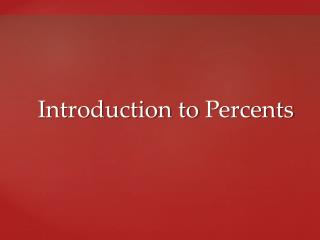DownloadDownload PresentationIntroduction to Percents

# Introduction to Percents

Télécharger la présentation## Introduction to Percents

- - - - - - - - - - - - - - - - - - - - - - - - - - - E N D - - - - - - - - - - - - - - - - - - - - - - - - - - -
##### Presentation Transcript

1. Introduction to Percents

2. Identify Terms Used With Percents • Percent • Like a fraction or a decimal, represents part of a whole. • Means hundredths or parts in 100 • Symbol is % • 25% = 25/100 = 0.25 (25 parts of 100 parts) • 33% = 33/100 = 0.33 (33 parts of 100 parts)

3. Write A Percent As A Decimal • Drop percent symbol and divide by 100 • 35% = 35/100 = 0.35 • Drop percent symbol and move decimal point 2 places to the left • 35% = 35.0 = 0.35

4. Let’s Try It • Convert the following percentages to decimals

5. Writing Mixed Number Percentages as Decimals • To write a mixed number percent as a decimal • Change the fractional part of the mixed number to a decimal percent. 8 ¼% = 8.25% • Convert decimal percent to its decimal equivalent • Move decimal point 2 places to the left 8.25% = 0.0825

6. Let’s Try It • Convert the following fractional percentages to decimals • Round numbers to the nearest hundredth

7. Common Fractions as Percents • 1/5 • Convert the fraction to its decimal equivalent 1/5 = 0.20 1 ÷ 5 = .20 • Convert the decimal to percent • Move decimal point 2 places to the right and add percent symbol 0.20 = 20%

8. Examples • To convert fractions to decimals • divide the numerator by the denominator

9. Let’s Try It

10. Let’s Try It

11. Independent Practice • Complete Worksheet 3.1 • #1 - 40

12. Part, Rate and Base

13. Percents are commonly used to determine interest, sales, taxes, commissions, and discounts or to make comparisons

14. Key Terms • Base • Represents 100% • That to which something is being compared • Rate • Number followed by percent symbol • The percent one number is of another one • May be written as decimal of fraction • Part • The number that is a portion of the base

15. As an Equation • Part = Base x Rate • As a proportion IS % = OF 100

16. For Example • What is a 30% discount on a \$150 jacket? • Equation: • Part = Base x Rate • ? = \$150 x 30% Convert to decimal and multiply \$150 x .30 = \$45

17. For Example • What is a 30% discount on a \$150 jacket? • Proportion Cross Multiply Divide both sides by 100 100X = (30)(150) 100X = 4500 100X = 4500 100 100 X IS % 30 = = 150 OF 100 100 X = \$45

18. Parts with decimals • What is 6.5% of 130? • Equation • Part = Base x Rate • X = 130 x .065 • X = 8.45

19. Parts With Decimals • What is a 6.5% of 130? • Proportion Cross Multiply Divide both sides by 100 100X = (6.5)(130) 100X = 845 100X = 845 100 100 X IS % 6.5 = = 130 OF 100 100 X = 8.45

20. Parts with Fractions • Suppose you had a gain of 6-1/4% on \$400. What was the gain? • Change fractional percent to a decimal percent 6.25% • Drop percent symbol and move decimal point to the left two spaces .0625 • Apply formula: Part = Base x Rate X = \$400 x .0625 X = \$25 • The gain was \$25

21. Finding Rate • Rate = Part ÷ Base Example: What percent is \$15 of \$139? X = 15 ÷ 139 X = 0.108 = 10.8%

22. For Example • What percent is \$15 of \$139? • Proportion Cross Multiply Divide both sides by 139 1500 = 139X 1500 = 139X 139 139 IS 15 X % = = 139 OF 100 100 X = 10.8

23. Independent Practice • Complete Worksheet 3.2A & 3.2B • #1 - 46